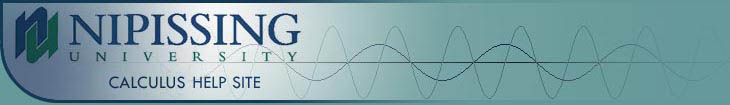TUTORIALS
TUTORIALS HOME

GENERAL MATH
NUMBER SETS
ABSOLUTE VALUE & INEQUALITIES
SETS & INTERVALS
FRACTIONS
POLYNOMIALS
LINEAR EQUATIONS
GEOMETRY
FINITE SERIES
TRIGONOMETRY
EXPONENTS
LOGARITHMS
INDUCTION

CALCULUS
LIMITS
DERIVATIVES
RELATED RATES & OPTIMIZATION
CURVE SKETCHING
INTEGRALS
AREA & VOLUME
INVERSE FUNCTIONS

MAIN
HOME
TESTS
TUTORIALS
SAMPLE PROBLEMS
COMMON MISTAKES
STUDY TIPS
GLOSSARY
CALCULUS APPLICATIONS
MATH HUMOUR

# Set Notation

A set is a collection of objects. Each object in the set is called an element of the set. If an element x is a member of the set S, we write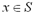. If an element x is not a member of the set S, we write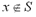. The empty set is the set that contains no elements. It is represented by {} or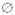. For sets A and B, A is called a subset of B, denoted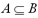, if every element of A is also an element of B.

The union of two sets A and B, denoted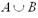is the set that consists of all elements that are in A or in B (or in both A and B). The intersection of two sets A and B, denoted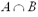is the set that consists of all elements that are in A and in B.

Sets are commonly defined in roster notation or set-builder notation. Roster notation provides a listing of each element in the set. For example, the set P of prime numbers between 0 and 10 (excluding 0 and 10) in roster notation is written as:

P = { 2, 3, 5, 7 }

The set-builder notation defines a general element of the set and then provides a ruling for each element in the set. The previous example, in set-builder notation is written as:

P = {x | x is prime and 0 < x < 10}

# Examples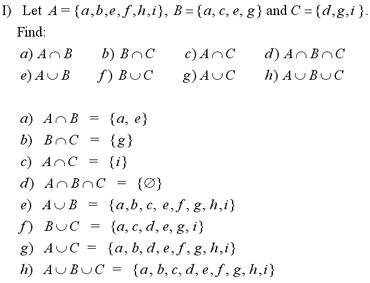Note: For another example of intersections and unions of sets, see question #1 in the Additional Examples section at the bottom of the page.

# The Real Number Line

Every real number can be associated with a single point on the real number line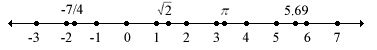# Intervals

An interval is a set that consists of all real numbers between a given pair of numbers. It can also be thought of as a segment of the real number line. An endpoint of an interval is either of the two points that mark the end of the line segment. An interval can include either endpoint, both endpoints or neither endpoint. To distinguish between these different intervals, we use interval notation.

An open interval does not include endpoints. The exclusion of the endpoints is indicated by round brackets () in interval notation. When the interval is represented by a segment of the real number line, the exclusion of an endpoint is illustrated by an open dot. For example, the interval of numbers between the integers 3 and 8, excluding 3 and 8, is written as

(3, 8) = {x | 3 < x < 8 }

in interval notation. As a segment of the real number line, it would be represented by the line below.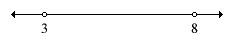A closed interval includes the endpoints. The inclusion of the endpoints is indicated by square brackets [] in interval notation. When the interval is represented by a segment of the real number line, the inclusion of an endpoint is illustrated by a closed dot. For example, the interval of numbers between the integers 1 and 11, including both 1 and 11, is written as

[1, 11] = {x | 1 £ x £ 11 }

in interval notation. As a segment of the real number line, it would be represented by the line below.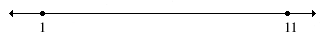One endpoint of an interval can be included, while the other is excluded. The interval [a, b) represents all numbers between a and b, including a but not b. Similarly, the interval (a, b] would represent all of the numbers between a and b, including b but not a. These intervals are shown in more detail in the table below.

Infinite intervals are those that do not have an endpoint in either the positive or negative direction, or both. The interval extends forever in that direction. Infinite intervals are summarized in the table below.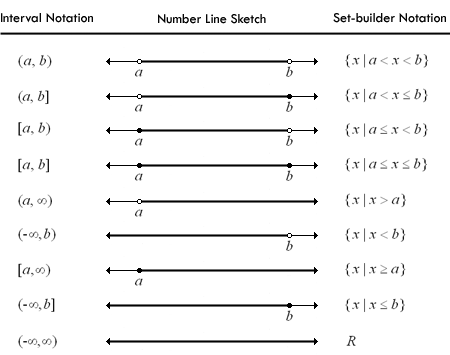Note: For an example involving intervals, see question #1 in the Additional Examples section below

1 | Example of the intersection and union of intervals

| Top of Page |

COURSE HOMEPAGES
MATH 1036
MATH 1037

FACULTY HOMEPAGES
Alex Karassev
Ted Chase